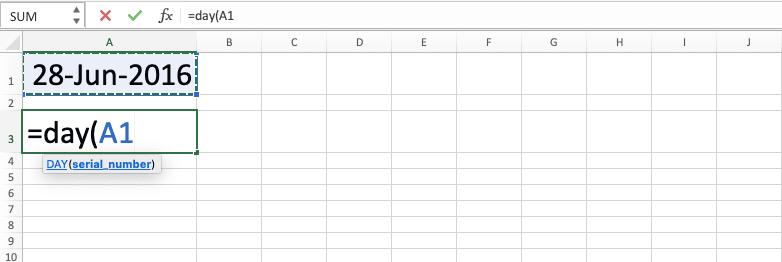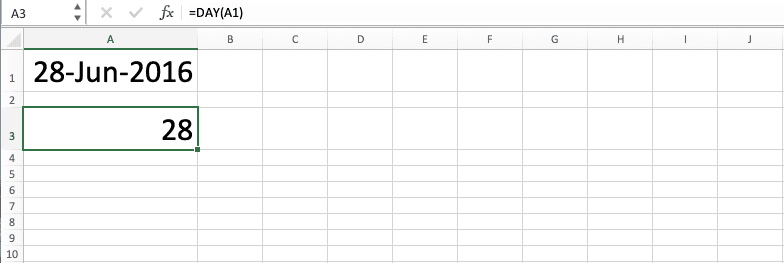How to Use the DAY Formula in Excel: Functions, Examples, and Writing Steps - Compute Expert

# How to Use the DAY Formula in Excel: Functions, Examples, and Writing Steps

Home >> Excel Tutorials from Compute Expert >> Excel Formulas List >> How to Use the DAY Formula in Excel: Functions, Examples, and Writing Steps

In this tutorial, you will learn how to use the DAY formula in excel completely.

When working with dates in excel, we may sometimes need to get the day number of our date. If we know the way to use the DAY formula in excel, then we can get that easily.

Want to know more about this DAY formula and understand the way to use it in excel? Read this tutorial until its last part!

Disclaimer: This post may contain affiliate links from which we earn commission from qualifying purchases/actions at no additional cost for you. Learn more

## What is the DAY Formula in Excel?

The DAY formula is a formula that can help us to get the day number of a date in excel.

## DAY Function in Excel

We can use DAY to get the day number of our date in excel. Just input the date we want to get the day number from to get its day number.

## DAY Result

The result we get from DAY is a number that represents the day number of the date we input into it.

## Excel Version from Which We Can Start Using DAY

We can start using DAY in excel since excel 2003.

## The Way to Write It and Its Input

Here is the general writing form of DAY in excel.

= DAY ( date )

The only input we need to give to DAY is the date we want to get our day number from.

## Example of Its Usage and Result

Here is a usage and result example of the DAY formula in excel.As you can see in the example, the way to use DAY in excel is actually quite easy. Just input the date we want to get our day number from to DAY and press enter. We will immediately get our result by doing that.

## Writing Steps

After we discussed the DAY writing form, input, and implementation example, now let’s discuss its writing steps. As DAY only needs a date input to run its function, the following writing steps should be easy to understand.

1. Type an equal sign ( = ) in the cell where you want to put the result in2. Type DAY (can be with large and small letters) and an open bracket sign after =3. Input the date you want to get the day number from4. Type a close bracket sign5. Press Enter
6. Done!## Get a Day Name from a Date: CHOOSE WEEKDAY

We may sometimes want to get the day name of our date instead of its day number. How can we do that easily in excel?

Unfortunately, it is hard to do that if we use DAY. The much easier method that we can utilize in excel is by combining the CHOOSE and WEEKDAY formulas.

Here is the general writing form of the CHOOSE and WEEKDAY combination for the day name retrieval purpose from our date.

= CHOOSE ( WEEKDAY ( date ) , “Sunday” , “Monday” , “Tuesday” , “Wednesday” , “Thursday” , “Friday” , “Saturday” )

We use WEEKDAY to get the day order of our date in its week, starting from Sunday to Saturday.

We write the WEEKDAY as our first CHOOSE input. Moreover, we input the day names from Sunday to Saturday as our CHOOSE result options.

By doing that, when we get our day order from WEEKDAY, it will determine what day name we get from CHOOSE. As a result, we will get the correct day name of our date from our CHOOSE and WEEKDAY combination!

To make you understand the formulas combination concept easier, here is its implementation example in excel.As you can see in the example, we can get the day name of our date by combining CHOOSE and WEEKDAY. We write the two formulas combination according to the way that we have discussed just now.

## Exercise

After you have learned how to use DAY in excel completely, you can practice your understanding by doing the exercise below!

### Questions

1. Fill column C with the day number of each date in column A!
2. Subtract the day number of each date in column B with the day number of the parallel date in column A. Fill the subtraction result in column D!
3. Subtract the day number of today’s date with the day number of each date in column A (use the TODAY formula to get today’s date). Fill the subtraction result in column E!

You can also get a day number result if you input a number from 1 to 2958465 to DAY. That is because a date is also equivalent to a specific number in excel.

Related tutorials you should learn from:

Get updated excel info from Compute Expert by registering your email. It's free!